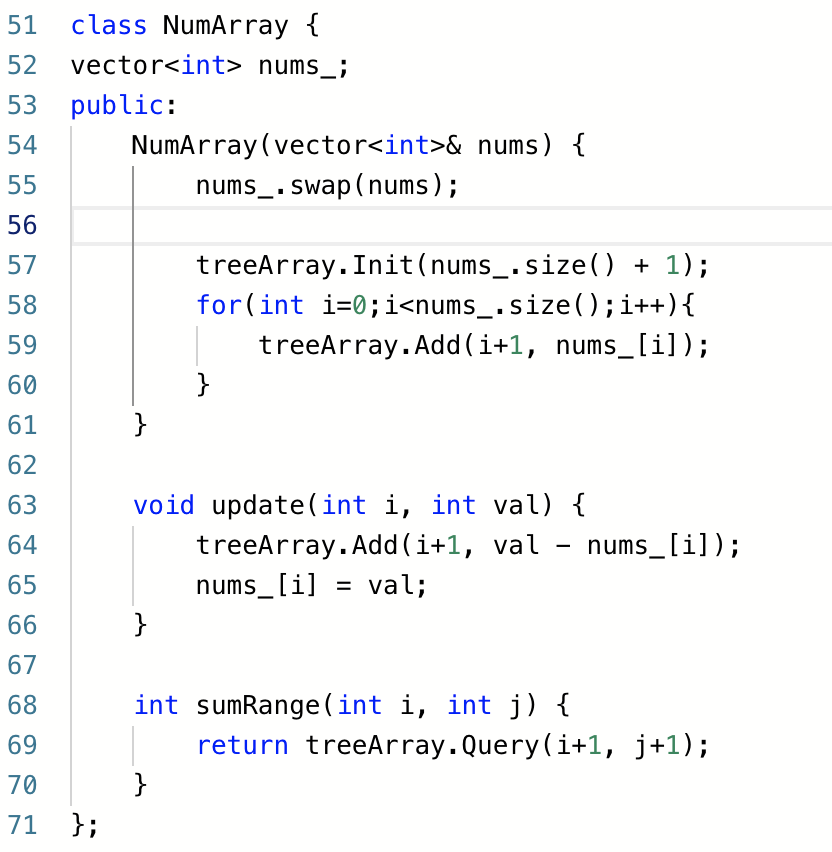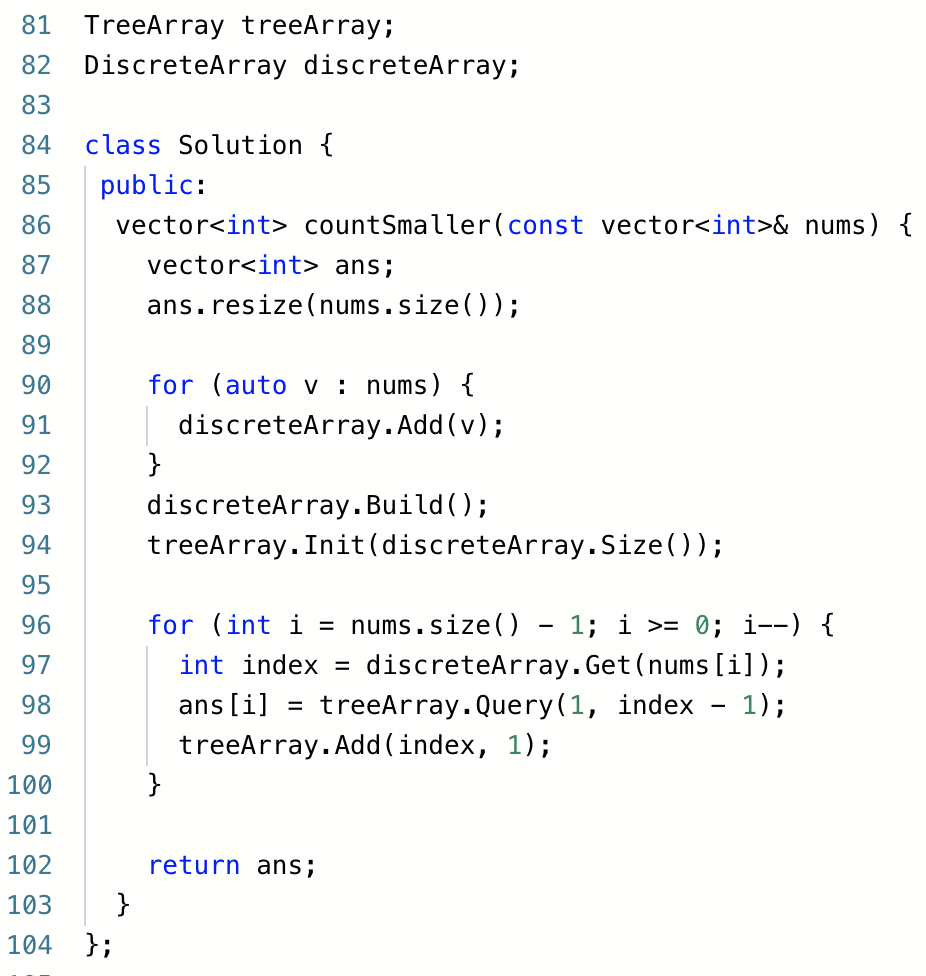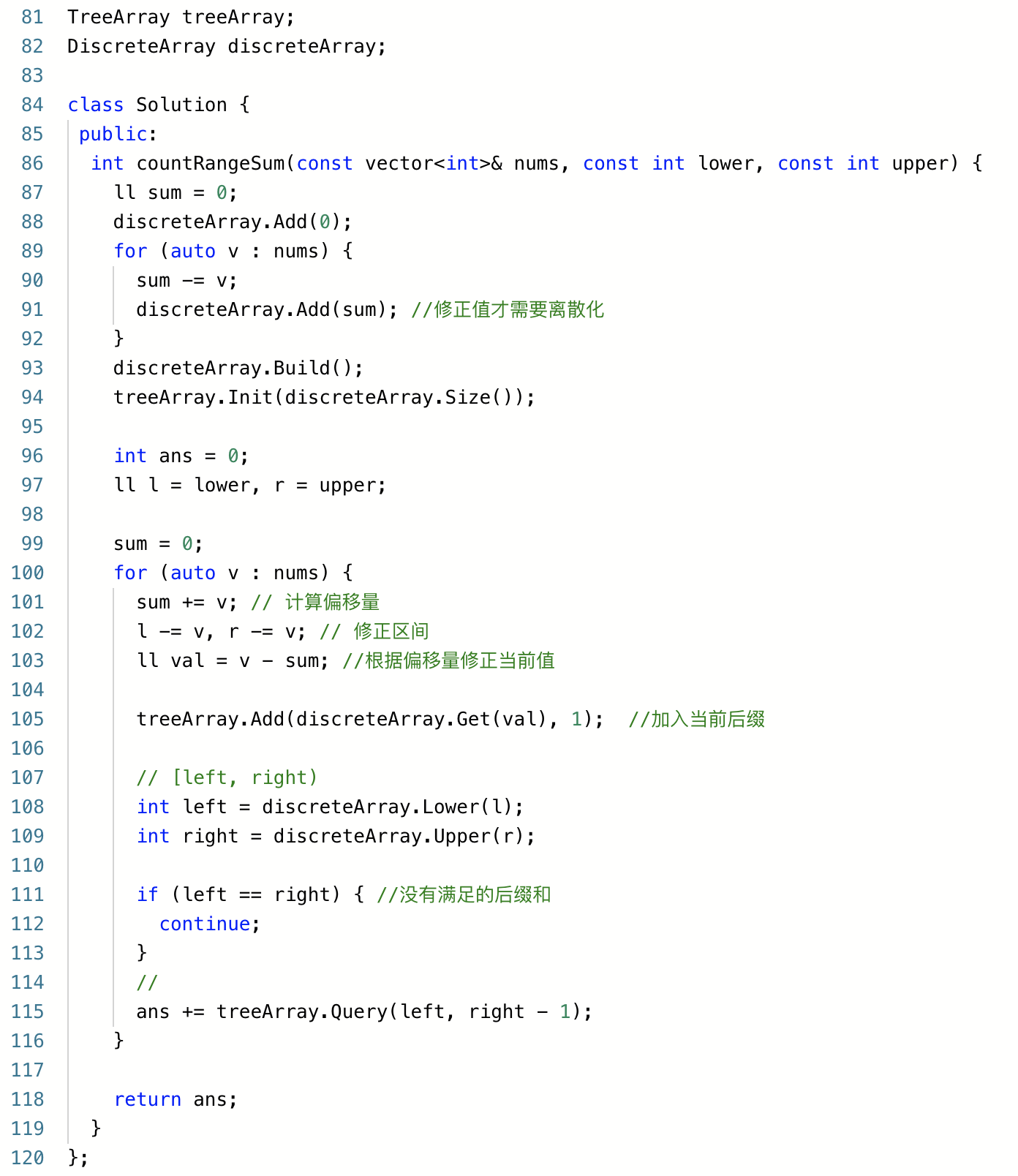# 初级树状数组 leetcode 练习题

## 二、区域和检索 - 数组可修改

2）修改下标 `i` 的值为`v`## 三、计算右侧小于当前元素的个数## 四、翻转对## 五、区间和的个数

``````sum[1  , j]
...
sum[j-2, j]
sum[j-1, j]
sum[j  , j]
``````

`j`的所有后缀和加上`nums[j+1]`，就几乎都是`j+1`的所有后缀和了。

``````sum[1  , j] + nums[j+1]
...
sum[j-2, j] + nums[j+1]
sum[j-1, j] + nums[j+1]
sum[j  , j] + nums[j+1]
nums[j+1]
``````《完》

-EOF-

## 关注公众号,接收最新消息

1.